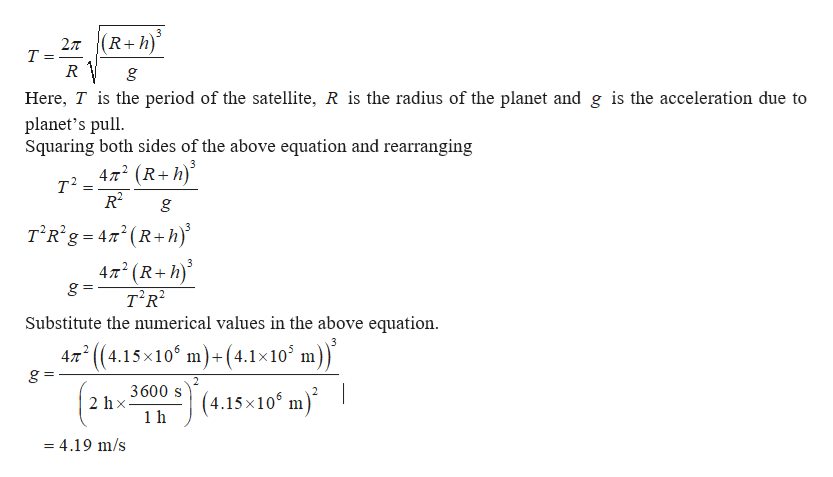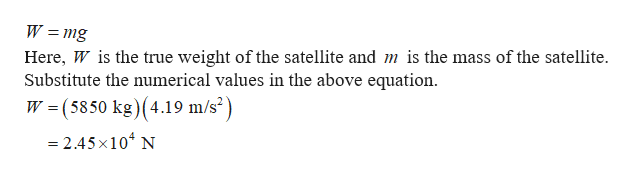The question states:A satellite has a mass of 5850 kg and is in a circular orbit 4.1 × 105 m above the surface of a planet. The period of the orbit is 2.00 hours. The radius of the planet is 4.15 × 106 m. What would be the true weight of the satellite if it were at rest on the planet's surface?I'm unsure as to how to draw the free body diagram as well as solving the problem. Any help would be aappreciated!

Question

The question states:A satellite has a mass of 5850 kg and is in a circular orbit 4.1 × 105 m above the surface of a planet. The period of the orbit is 2.00 hours. The radius of the planet is 4.15 × 106 m. What would be the true weight of the satellite if it were at rest on the planet's surface?

I'm unsure as to how to draw the free body diagram as well as solving the problem. Any help would be aappreciated!

Step 1

The equation for the time period of the satellite is given byhelp_outlineImage Transcriptionclose27 (R+h) т g Here, T is the period of the satellite, R is the radius of the planet and g is the acceleration due to planet's pull Squaring both sides of the above equation and rearranging 472 (R+h т? T Rig 42R+h) 472 (R+h) g = т'R? Substitute the numerical values in the above equation - (4.15x10 m) +(4.1x 10 m) 2 47T g 2 3600s (4.15x1061 2hx m = 4.19 m/s fullscreen
Step 2

The equation for the true weight o...help_outlineImage TranscriptioncloseW mg Here, W is the true weight of the satellite and m is the mass of the satellite Substitute the numerical values in the above equation W (5850 kg) (4.19 m/s2) - 2.45x104 N fullscreen

Want to see the full answer?

See Solution

Want to see this answer and more?

Our solutions are written by experts, many with advanced degrees, and available 24/7

See Solution
Tagged in

Gravitation